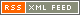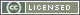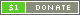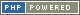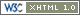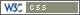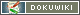# Numerical Operators

Number Operators perform various operations on the terms specified. There are several different types of operation available.

Symbol Type Name Description Example
Arithmetic
+ Infix Addition Adds the right term to the left term to give the sum ` a + b `
- Infix Subtraction Takes the right term away from the left term to give the difference ` a - b `
* Infix Multiplication Multiplies the left term by the right term to give the product ` a * b `
/ Infix Division Divides the left term by the right term to give the quotient ` a / b `
% Infix Modulus Divides the left term by the right term and gives the remainder ` a % b `
** Infix Exponentiation Raises the left term to the power specified by the right term ` a ** b `
Auto-Increment/Auto-Decrement
++ Prefix Pre-increment Increments the term by 1, then returns the new value ` ++a `
++ Postfix Post-increment Returns the term value, then increments the term by 1 ` a++ `
-- Prefix Pre-decrement Decrements the term by 1, then returns the new value ` --a `
-- Postfix Post-decrement Returns the term value, then decrements the term by 1 ` a-- `
Bitwise
+& Infix AND Bits that are set in both left and right terms are set ` a +& b `
+| Infix OR Bits that are set in either left or right terms are set ` a +| b `
+^ Infix XOR Bits that are set in either left or right terms, but not both, are set ` a +^ b `
+^ Prefix NOT All bits in the term are inverted ` +^ a `
+< Infix Shift left Shift the bits of the left term left by the number of steps specified in the right term ` a +< b `
+> Infix Shift right Shift the bits of the left term right by the number of steps specified in the right term ` a +> b `
Mathematical
abs Prefix Absolute value Gives the positive numerical value of the term ` abs a `
sin Prefix Sine Gives the sine of the term, with the term in radians ` sin a `
cos Prefix Cosine Gives the cosine of the term, with the term in radians ` cos a `
tan Prefix Tangent Gives the tangent of the term, with the term in radians ` tan a `
log Prefix Logarithm Gives the base-10 logarithm of the term ` log a `
ln Prefix Natural logarithm Gives the natural logarithm of the term ` ln a `
Type Casting
+ Prefix Cast Converts the term to a Number, without affecting the sign ` +a `
- Prefix Inverse cast Converts the term to a Number, and inverts the sign ` -a `
Type Checking
is Infix IsType Checks if the value of the left term is of the type specified by the right term `a is number`

Each Operator will evaluate the terms provided as appropriate to the Operator value type - for instance, performing a numerical addition on two Strings will convert the Strings to Numbers first.